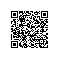# Linux命令之awk

awk 中输出双引号和单引号

awk '{print "\""}'
awk '{print "'\''"}'


awk -F "," '{if($(NF-1)=="2"){print$0}}' | wc -l

awk -F "," ' BEGIN { count1=0;count2=0;count3=0} { if((NF-1)==1){count1+=1} else if((NF-1)==2){count2+=1} else{count3+=1} } END {print NR,count1,count2,count3} ' 20151120.txt


awk 取整行 $0，取第一列$1，取最后一列 $NF，取倒数第二列$(NF-1)使用钉钉扫一扫加入圈子
+ 订阅